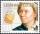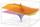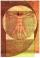# Pumps

The tank is filled with two pumps in 16 minutes. The first pump is filled in 30 minutes earlier than two one. How many minutes is filled with the first pump?

Result

t1 =  22.9 min

#### Solution:Leave us a comment of example and its solution (i.e. if it is still somewhat unclear...):Be the first to comment!#### To solve this verbal math problem are needed these knowledge from mathematics:

Looking for help with calculating roots of a quadratic equation? Do you have a linear equation or system of equations and looking for its solution? Or do you have quadratic equation?

## Next similar examples:

1. PoolIf water flows into the pool by two inlets, fill the whole for 8 hours. The first inlet filled pool 6 hour longer than second. How long pool take to fill with two inlets separately?
2. CuboidCuboid with edge a=16 cm and body diagonal u=45 cm has volume V=11840 cm3. Calculate the length of the other edges.
3. TrainsFrom station 130 km away started passenger train and after 2.2 hours after the express train, which travels 37 km an hour more. Express train finish journey 7 minutes early. Calculate the average speed of this two trains.
4. GP - three membersThe second and third of a geometric progression are 24 and 12(c+1) respectively, given that the sum of the first three terms of progression is 76 determine value of c
5. R triangleCalculate the area of a right triangle whose longer leg is 6 dm shorter than the hypotenuse and 3 dm longer than the shorter leg.
6. The hallThe hall had a rectangular ground plan one dimension 20 m longer than the other. After rebuilding the length of the hall declined by 5 m and the width has increased by 10 m. Floor area increased by 300 m2. What were the original dimensions of the hall?
7. Touch x-axisFind the equations of circles that pass through points A (-2; 4) and B (0; 2) and touch the x-axis.
8. Right triangle AlefThe obvod of a right triangle is 84 cm, the hypotenuse is 37 cm long. Determine the lengths of the legs.
9. Right triangleLegs of right are in ratio a:b = 2:8. Hypotenuse has a length of 87 cm. Calculate the perimeter and area of the triangle.
10. Rectangle SSPerimeter of a rectangle is 268 cm and its diagonal is 99.3 cm. Determine the dimensions of the rectangle.
11. RT - hypotenuse and altitudeRight triangle BTG has hypotenuse g=117 m and altitude to g is 54 m. How long are hypotenuse segments?
12. CircleCircle touch two parallel lines p and q; and its center lies on a line a, which is secant of lines p and q. Write the equation of circle and determine the coordinates of the center and radius. p: x-10 = 0 q: -x-19 = 0 a: 9x-4y+5 = 0
13. Trapezoidtrapezoid ABCD a = 35 m, b=28 m c = 11 m and d = 14 m. How to calculate its area?
14. Euler problemSomeone buys a 180 tolars towels. If it was for the same money of 3 more towels, it would be 3 tolars cheaper each. How many were towels?
15. SequenceIn the arithmetic sequence is given: Sn=2304, d=2, an=95 Calculate a1 and n.
16. Sum-logThe sum of two numbers is 32, the sum of their logarithms (base 10) is 2.2. Determine these numbers.
17. Golden ratioDivide line of length 14 cm into two sections that the ratio of shorter to greater is same as ratio of greater section to whole length of the line.